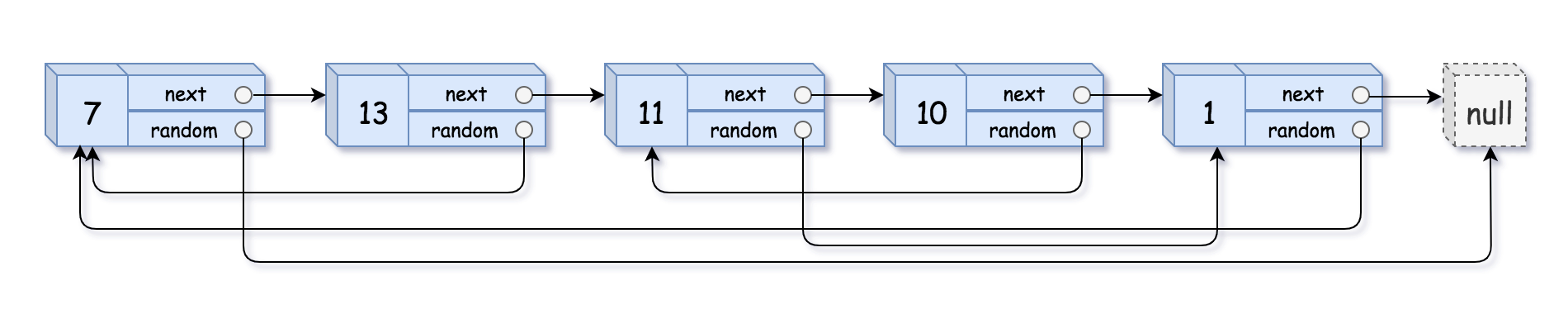# 138. Copy List with Random Pointer | LeetCode Solution

## 138. Copy List with Random Pointer | LeetCode Solution

A linked list of length `n` is given such that each node contains an additional random pointer, which could point to any node in the list, or `null`.

Construct a deep copy of the list. The deep copy should consist of exactly `n` brand new nodes, where each new node has its value set to the value of its corresponding original node. Both the `next` and `random` pointer of the new nodes should point to new nodes in the copied list such that the pointers in the original list and copied list represent the same list state. None of the pointers in the new list should point to nodes in the original list.

For example, if there are two nodes `X` and `Y` in the original list, where `X.random --> Y`, then for the corresponding two nodes `x` and `y` in the copied list, `x.random --> y`.

The linked list is represented in the input/output as a list of `n` nodes. Each node is represented as a pair of `[val, random_index]` where:

• `val`: an integer representing `Node.val`
• `random_index`: the index of the node (range from `0` to `n-1`) that the `random` pointer points to, or `null` if it does not point to any node.

Your code will only be given the `head` of the original linked list.

Example 1:```Input: head = [[7,null],[13,0],[11,4],[10,2],[1,0]]
Output: [[7,null],[13,0],[11,4],[10,2],[1,0]]
```

Example 2:```Input: head = [[1,1],[2,1]]
Output: [[1,1],[2,1]]
```

Example 3:```Input: head = [[3,null],[3,0],[3,null]]
Output: [[3,null],[3,0],[3,null]]
```

Constraints:

• `0 <= n <= 1000`
• `-104 <= Node.val <= 104`
• `Node.random` is `null` or is pointing to some node in the linked list.

/*
// Definition for a Node.
class Node {
public:
int val;
Node* next;
Node* random;

Node(int _val) {
val = _val;
next = NULL;
random = NULL;
}
};
*/

class Solution {
public:
map<Node*, Node*> mapped_node;

return NULL;